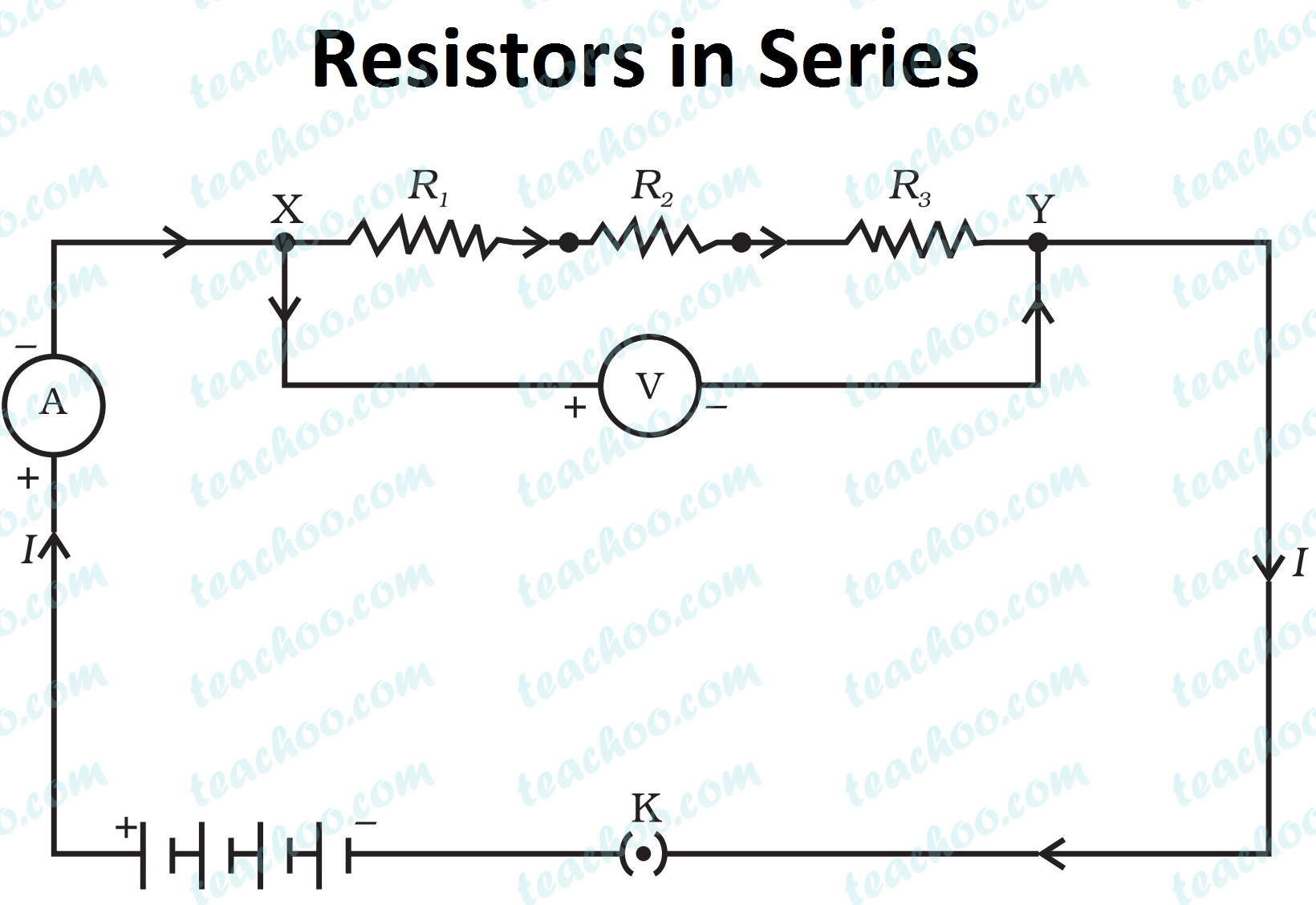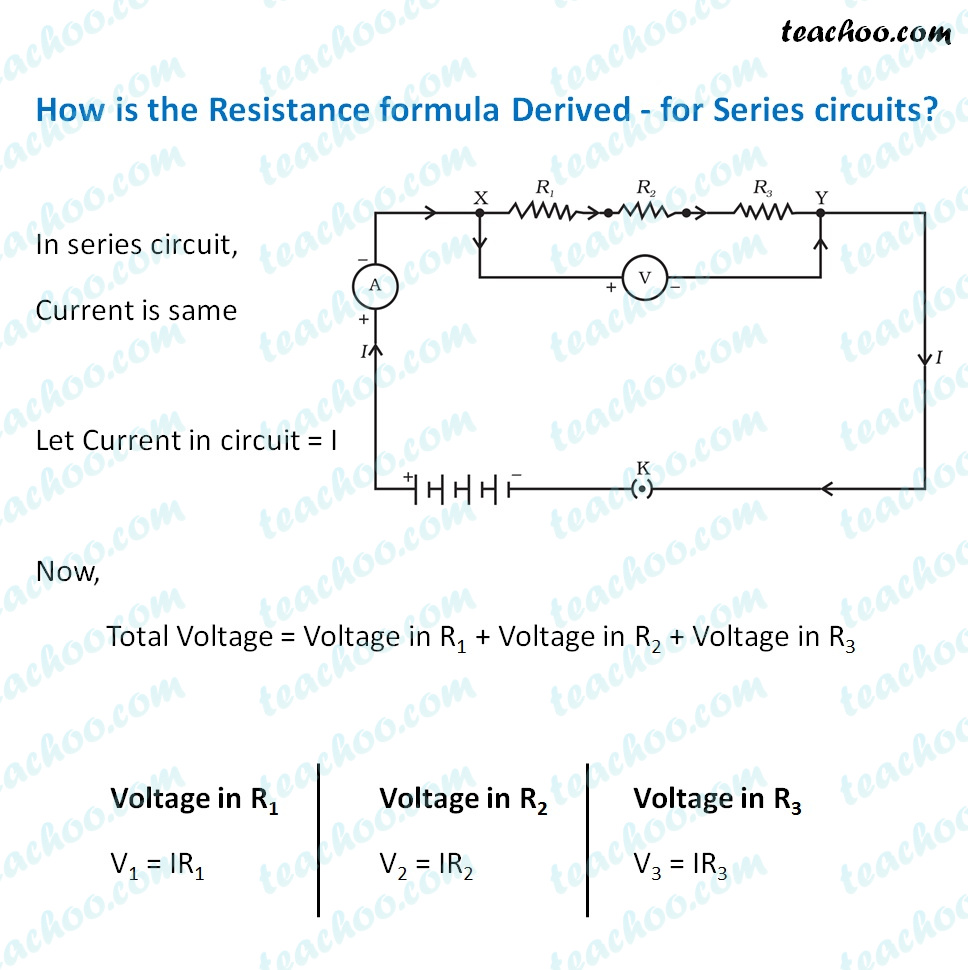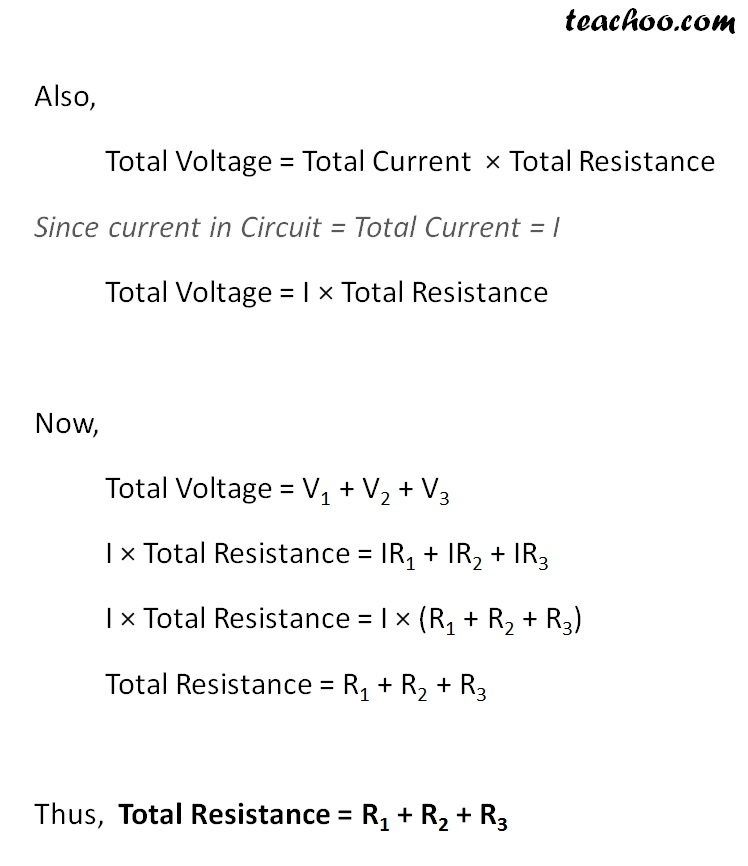Concepts

Class 10
Chapter 12 Class 10 - Electricity

In this type of combination, different resistors are joined end to end. (one after the another)

ExampleThis is done to Increase Resistance of Circuit

In this case Total Resistance of circuit is equal to sum of Individual Resistance of the Resistors

R = R 1 + R 2 + R 3

Example

Suppose Resistance of 2 resistors are 20 Ω and 30 Ω

Hence,R 1 = 20 Ω and R 2 = 30 Ω

Total Resistance = R 1 + R 2 = 20 + 30 = 50 Ω

## IMPORTANT POINTS

### When 2 Resistors are Connected in Series

1. Same Current flows throughout the circuit in all resistors Let it be denoted by I

2. Potential Difference across combination of Resistors is equal to total Potential Difference of Each Resistor
Suppose 3 Different Resistors have Potential Difference of V 1 , V 2 and V 3
Total Potential Difference V = V 1 + V 2 +V 3
Hence,Total Potential Difference is equal to voltage of the battery

3. Total Resistance is equal to Individual Resistance of each Resistor Hence R = R 1 + R 2 + R 3

### How is the Resistance formula Derived - for series circuits?Resistance of first resistor+second resistor+third resistor = Total Resistance

Hence we can say that sum of Individual Resistance of 3 resistors is equal to Total Resistance

So more than one resistors are combined together to increase resistance of the circuit

More the Resistance,less the current flows through circuit

Hence,this method is used to reduce current flow in circuit

Note - : In case of series, the total resistance is always greater than the resistance of the resistors.
The resistance produced is highest when resistors are connected in series.

Note - : An ammeter is connected in series in a circuit so that it can detect the net current flowing in a circuit.

## Questions

Q1 Page 213 - Draw a schematic diagram of a circuit consisting of a battery of three cells of 2V each , a 5Ω resistor, an 8 Ω resistor, and a 12 Ω resistor. And a plug key, all connected in series.

Q2 Page 213 - Redraw the circuit of Question 1, putting in an ammeter to measure the current through the resistors and a voltmeter to measure the potential difference across the 12 Ω resistor. What would be the readings in the ammeter and the voltmeter?

Example 12.7 - An electric lamp, whose resistance is 20 Ω, and a conductor of 4 Ω resistance are connected to a 6 V battery (Fig. 12.9). Calculate
(a) the total resistance of the circuit,
(b) the current through the circuit, and
(c) the potential difference across the electric lamp and conductor.

Question 4 - What is the maximum resistance which can be made using five

(a) 1/5 Ω

(b) 10 Ω

(c) 5 Ω

(d) 1 Ω

Question 9 - A cell, a resistor, a key and ammeter are arranged as shown in the circuit diagrams below. The current recorded in the ammeter will be

(a) maximum in (i)

(b) maximum in (ii)

(c) maximum in (iii)

(d) the same in all the cases

Question 11 - Why does Ammeter have low resistance? Given reason

Why does Voltmeter have high resistance?

Learn in your speed, with individual attention - Teachoo Maths 1-on-1 Class

### Transcript

How is the Resistance formula Derived - for Series circuits? In series circuit, Current is same Let Current in circuit = I Now, Total Voltage = Voltage in R1 + Voltage in R2 + Voltage in R3 Voltage in R1 V1 = IR1 Voltage in R2 V2 = IR2 Voltage in R3 V3 = IR3 Also, Total Voltage = Total Current × Total Resistance Since current in Circuit = Total Current = I Total Voltage = I × Total Resistance Now, Total Voltage = V1 + V2 + V3 I × Total Resistance = IR1 + IR2 + IR3 I × Total Resistance = I × (R1 + R2 + R3) Total Resistance = R1 + R2 + R3 Thus, Total Resistance = R1 + R2 + R3